# Chemical reactionsPage 1

#### WATCH ALL SLIDES

Slide 1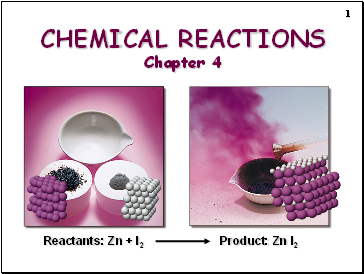CHEMICAL REACTIONS Chapter 4

Reactants: Zn + I2

Product: Zn I2

Slide 2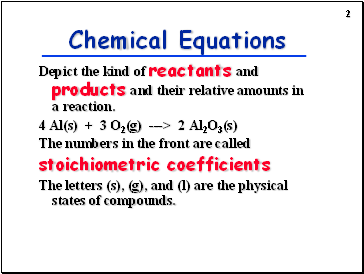## Chemical Equations

Depict the kind of reactants and products and their relative amounts in a reaction.

4 Al(s) + 3 O2(g) ---> 2 Al2O3(s)

The numbers in the front are called

stoichiometric coefficients

The letters (s), (g), and (l) are the physical states of compounds.

Slide 3Chemical Equations

4 Al(s) + 3 O2(g) ---> 2 Al2O3(s)

This equation means

4 Al atoms + 3 O2 molecules ---give--->

2 molecules of Al2O3

4 moles of Al + 3 moles of O2 ---give--->

2 moles of Al2O3

Slide 4Chemical Equations

Because the same atoms are present in a reaction at the beginning and at the end, the amount of matter in a system does not change.

The Law of the Conservation of Matter

Demo of conservation of matter, See Screen 4.3.

Slide 5Because of the principle of the conservation of matter,

an equation must be balanced.

It must have the same number of atoms of the same kind on both sides.

Chemical Equations

Slide 6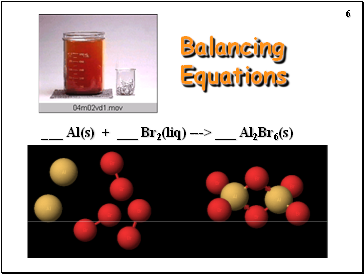## Balancing Equations

_ Al(s) + _ Br2(liq) ---> _ Al2Br6(s)

Slide 7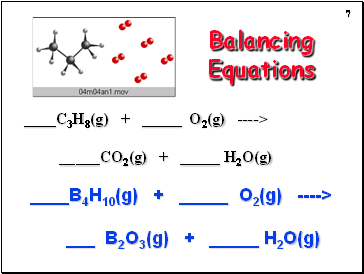Balancing Equations

C3H8(g) + _ O2(g) ----> _CO2(g) + _ H2O(g)

B4H10(g) + _ O2(g) ----> _ B2O3(g) + _ H2O(g)

Slide 8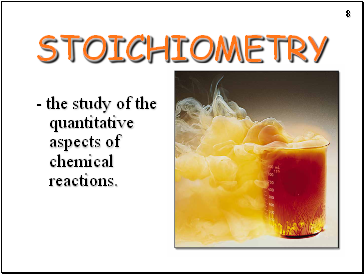## Stoichiometry

- the study of the quantitative aspects of chemical reactions.

Slide 9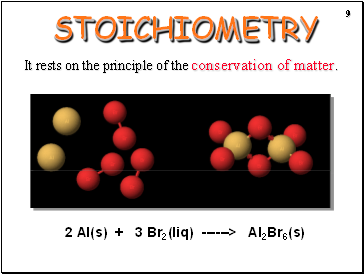STOICHIOMETRY

It rests on the principle of the conservation of matter.

2 Al(s) + 3 Br2(liq) ------> Al2Br6(s)

Slide 10PROBLEM: If 454 g of NH4NO3 decomposes, how much N2O and H2O are formed? What is the theoretical yield of products?

STEP 1

Write the balanced chemical equation

NH4NO3 ---> N2O + 2 H2O

Slide 11454 g of NH4NO3 --> N2O + 2 H2O

STEP 2 Convert mass reactant (454 g) --> moles

STEP 3 Convert moles reactant (5.68 mol) --> moles product

Slide 12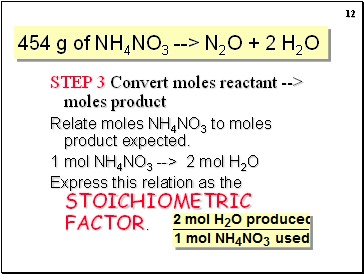Go to page:
1  2  3  4